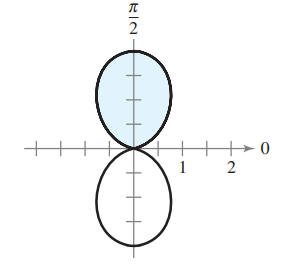×
Get Full Access to Calculus: Early Transcendental Functions - 6 Edition - Chapter 10.5 - Problem 4
Get Full Access to Calculus: Early Transcendental Functions - 6 Edition - Chapter 10.5 - Problem 4

×

# ?In Exercises 1-4, write an integral that represents the area of the shaded region of the figure. Do not evaluate the integral. $$r=1-\cos 2 \ISBN: 9781285774770 141 ## Solution for problem 4 Chapter 10.5 Calculus: Early Transcendental Functions | 6th Edition • Textbook Solutions • 2901 Step-by-step solutions solved by professors and subject experts • Get 24/7 help from StudySoup virtual teaching assistantsCalculus: Early Transcendental Functions | 6th Edition 4 5 1 412 Reviews 29 2 Problem 4 In Exercises 1-4, write an integral that represents the area of the shaded region of the figure. Do not evaluate the integral. \(r=1-\cos 2 \theta$$Text Transcription:

r = 1 - cos 2 theta

Step-by-Step Solution:

Step 1 of 5) L’Hôpital’s Rule applies to one-sided limits as well. Example 3 In this example the one-sided limits are different.

Step 2 of 2

##### ISBN: 9781285774770

Since the solution to 4 from 10.5 chapter was answered, more than 225 students have viewed the full step-by-step answer. The answer to “?In Exercises 1-4, write an integral that represents the area of the shaded region of the figure. Do not evaluate the integral.$$r=1-\cos 2 \theta$$ Text Transcription:r = 1 - cos 2 theta” is broken down into a number of easy to follow steps, and 32 words. Calculus: Early Transcendental Functions was written by and is associated to the ISBN: 9781285774770. This full solution covers the following key subjects: . This expansive textbook survival guide covers 134 chapters, and 10738 solutions. The full step-by-step solution to problem: 4 from chapter: 10.5 was answered by , our top Calculus solution expert on 11/14/17, 10:53PM. This textbook survival guide was created for the textbook: Calculus: Early Transcendental Functions, edition: 6.

## Discover and learn what students are asking

Unlock Textbook Solution